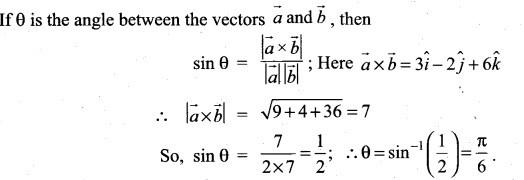# Samacheer Kalvi 11th Maths Solutions Chapter 8 Vector Algebra – I Ex 8.4

## Tamilnadu Samacheer Kalvi 11th Maths Solutions Chapter 8 Vector Algebra – I Ex 8.4

Question 1.
Find the magnitude of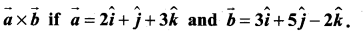Solution: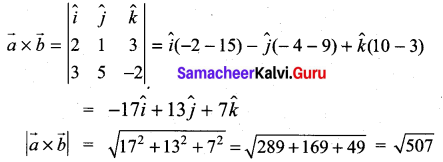Question 2.
Show that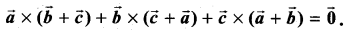Solution: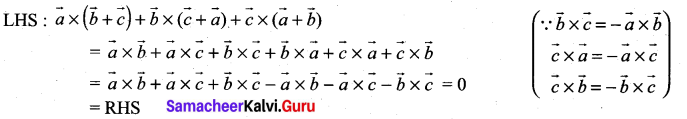Question 3.
Find the vectors of magnitude $$10 \sqrt{3}$$ that are perpendicular to the plane which contains $$\hat{i}+2 \hat{j}+\hat{k}$$ and $$\hat{i}+3 \hat{j}+4 \hat{k}$$
Solution: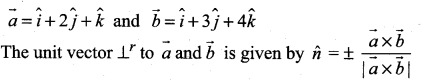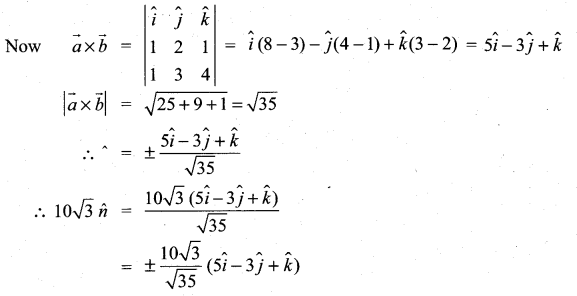Question 4.
Find the unit vectors perpendicular to each of the vectors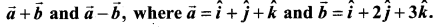Solution: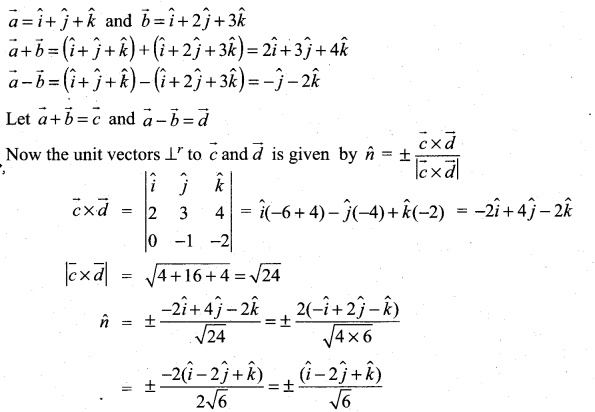Question 5.
Find the area of the parallelogram whose two adjacent sides are determined by the vectors $$\hat{i}+2 \hat{j}+3 \hat{k}$$ and $$3 \hat{i}-2 \hat{j}+\hat{k}$$
Solution: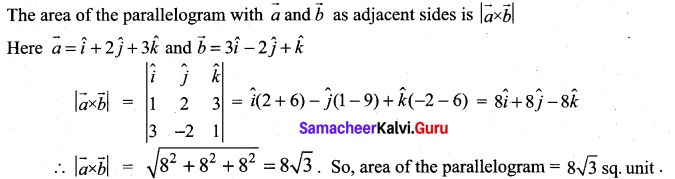Question 6.
Find the area of the triangle whose vertices are A(3, -1, 2), B(1, -1, -3) and C(4, -3, 1)
Solution:
A = (3, -1, 2); B = (1, -1, -3) and C = (4, -3, 1)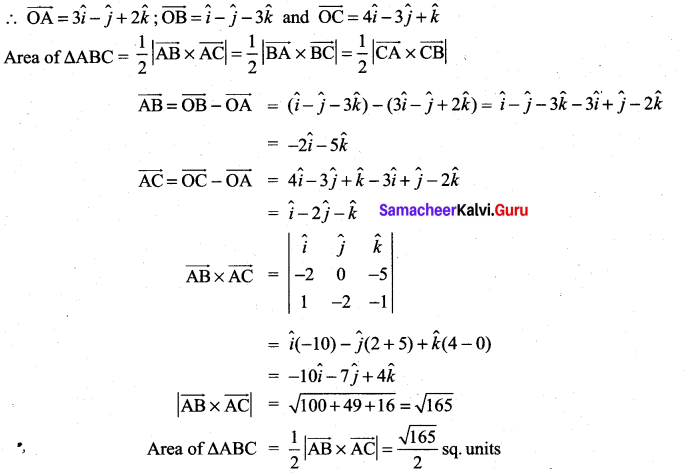Question 7.
If $$\vec{a}, \vec{b}, \vec{c}$$ are position vectors of the vertices A, B, C of a triangle ABC, show that the area of the triangle ABC is $$\frac{1}{2}|\vec{a} \times \vec{b}+\vec{b} \times \vec{c}+\vec{c} \times \vec{a}|$$. Also deduce the condition for collinearity of the points A, B, C
Solution: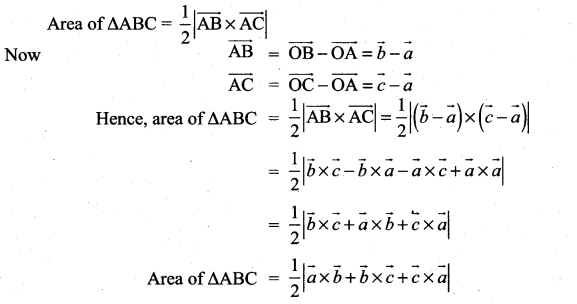If the points A, B, C are collinear, then the area of ∆ABC = 0.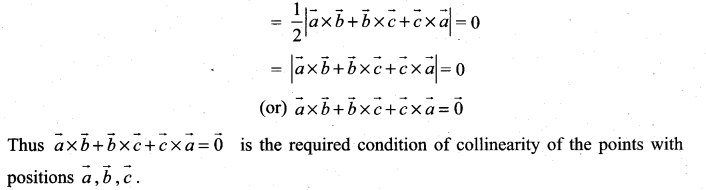Question 8.
For any vector $$\vec{a}$$ prove that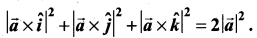Solution: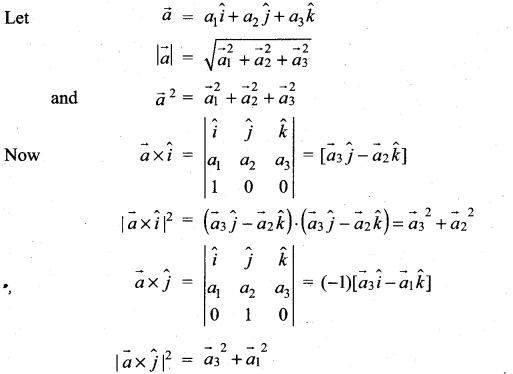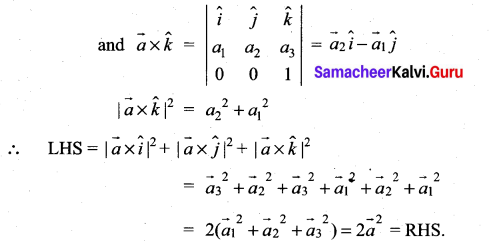Question 9.
Let $$\vec{a}, \vec{b}, \vec{c}$$ be unit vectors such that $$\overrightarrow{\boldsymbol{a}} \cdot \overrightarrow{\boldsymbol{b}}=\overrightarrow{\boldsymbol{a}} \cdot \overrightarrow{\boldsymbol{c}}=\mathbf{0}$$ and the angle between $$\vec{b} \text { and } \vec{c} \text { is } \frac{\pi}{3}$$. Prove that $$\vec{a}=\pm \frac{2}{\sqrt{3}}(\vec{b} \times \vec{c})$$
Solution:
Given $$|\vec{a}|=|\vec{b}|=|\vec{c}|$$ = 1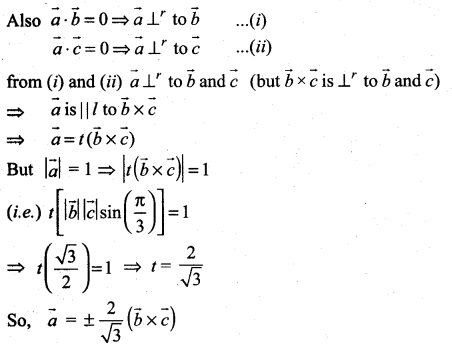Question 10.
Find the angle between the vectors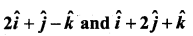using vector product
Solution:
The angle between $$\vec{a}$$ and $$\vec{b}$$ using vector product is given by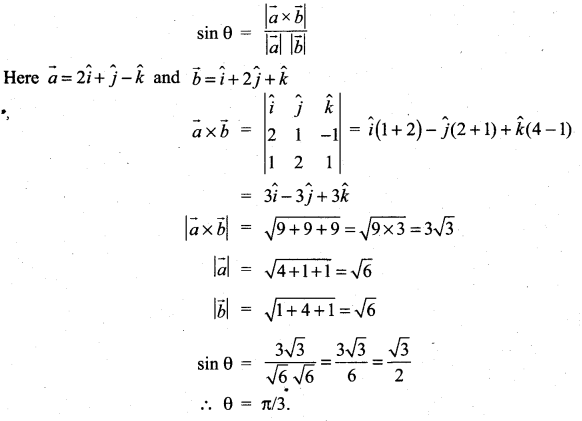### Samacheer Kalvi 11th Maths Solutions Chapter 8 Vector Algebra – I Ex 8.4 Additional Problems

Question 1.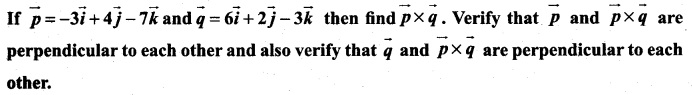Solution: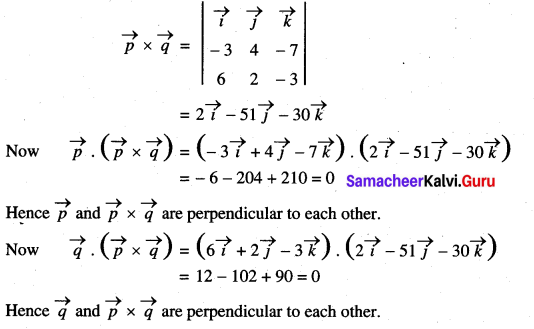Question 2.
If $$\vec{a}$$, $$\vec{b}$$ are any two vectors, then prove that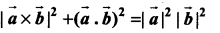Solution: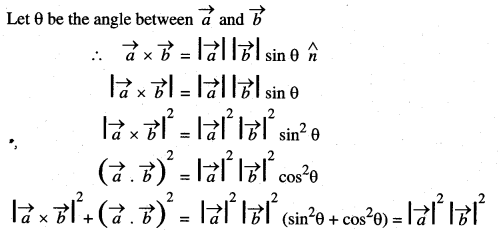Question 3.
Find the angle between the vectors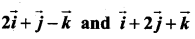by using cross product.
Solution: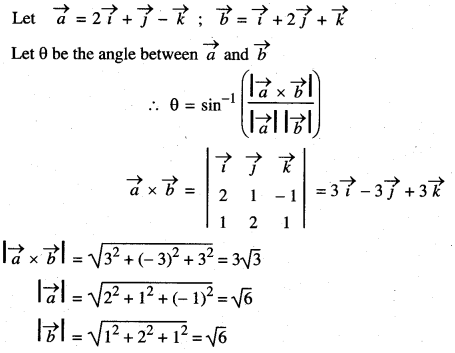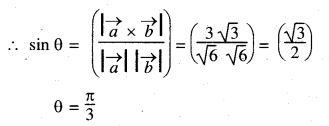Question 4.
Find the vector of magnitude 6 which are perpendicular to both the vectors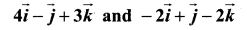Solution: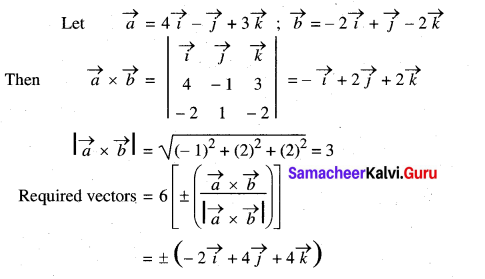Question 5.
Find the vectors whose length 5 which are perpendicular to the vectors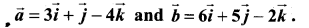Solution: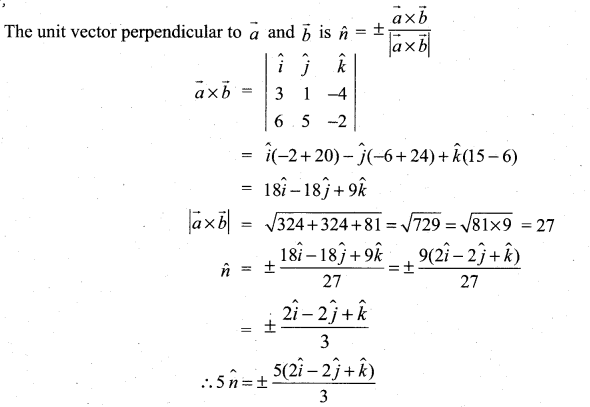Question 6.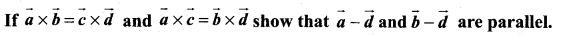Solution:
Given $$\vec{a} \times \vec{b}=\vec{c} \times \vec{d}$$ and $$\vec{a} \times \vec{c}=\vec{b} \times \vec{d}$$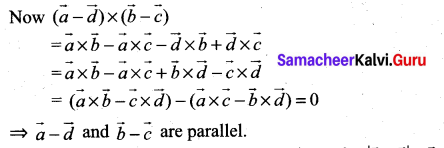Question 7.
Find the angle between two vectors $$\vec{a}$$ and $$\vec{b}$$ if $$|\overrightarrow{\boldsymbol{a}} \times \overrightarrow{\boldsymbol{b}}|=\overrightarrow{\boldsymbol{a}} \cdot \overrightarrow{\boldsymbol{b}}$$
Solution: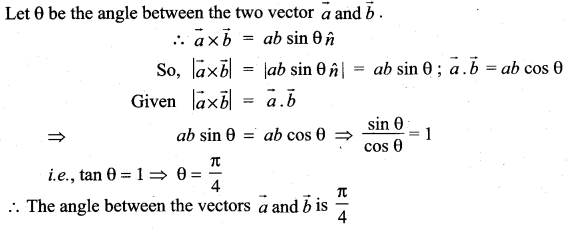Question 8.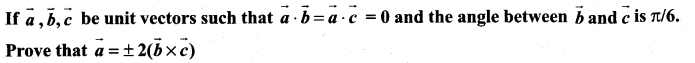Solution: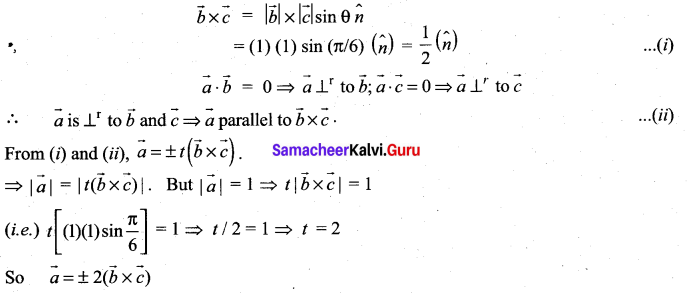Question 9.
If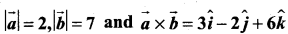find the angle between $$\vec{a}$$ and $$\vec{b}$$
Solution: